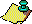Contents Next: Connections of the Up: The 3x+1 problem. Previous: Are there non-trivial

Several authors have observed that heuristic probabilistic arguments suggest that no divergent trajectories occur.

### DIVERGENT TRAJECTORIES CONJECTURE.

The function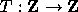has no divergent trajectories, i.e., there exists no integer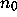for which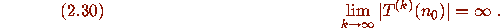If a divergent trajectory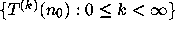exists, it cannot be equidistributed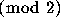. Indeed if one defines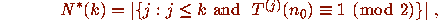then it can be proved that the condition (2.30) implies thatTheorem F constrains the possible behavior of divergent trajectories. Indeed, associated to any divergent trajectory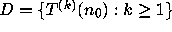is the infinite set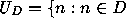and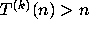for all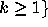. Since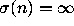for all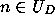, Theorem F implies that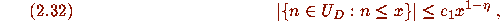where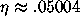. Roughly speaking, (2.32) asserts that the elements of a divergent trajectory cannot go to infinity ``too slowly.''Contents Next: Connections of the Up: The 3x+1 problem. Previous: Are there non-trivial